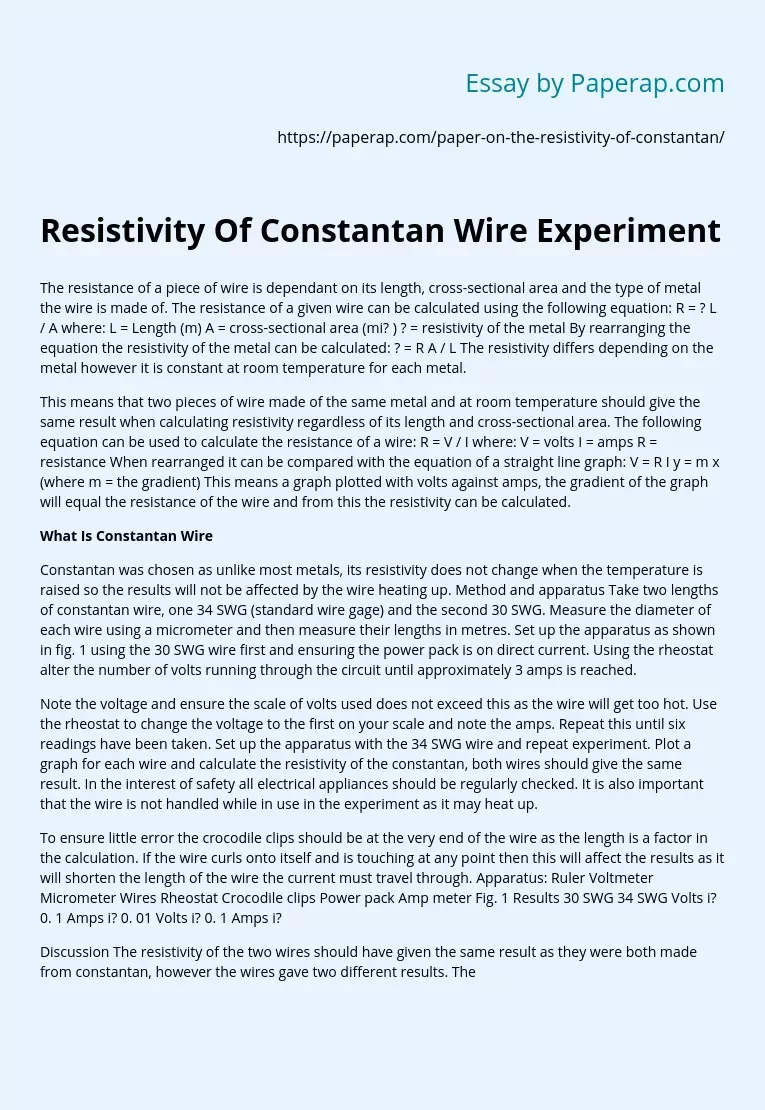# Resistivity Of Constantan Wire Experiment

The resistance of a piece of wire is dependant on its length, cross-sectional area and the type of metal the wire is made of. The resistance of a given wire can be calculated using the following equation: R = ? L / A where: L = Length (m) A = cross-sectional area (mi? ) ? = resistivity of the metal By rearranging the equation the resistivity of the metal can be calculated: ? = R A / L The resistivity differs depending on the metal however it is constant at room temperature for each metal.

This means that two pieces of wire made of the same metal and at room temperature should give the same result when calculating resistivity regardless of its length and cross-sectional area. The following equation can be used to calculate the resistance of a wire: R = V / I where: V = volts I = amps R = resistance When rearranged it can be compared with the equation of a straight line graph: V = R I y = m x (where m = the gradient) This means a graph plotted with volts against amps, the gradient of the graph will equal the resistance of the wire and from this the resistivity can be calculated.

## What Is Constantan Wire

Constantan was chosen as unlike most metals, its resistivity does not change when the temperature is raised so the results will not be affected by the wire heating up. Method and apparatus Take two lengths of constantan wire, one 34 SWG (standard wire gage) and the second 30 SWG. Measure the diameter of each wire using a micrometer and then measure their lengths in metres.

Get quality help nowWriter LylaVerified

Proficient in: Electrical Engineering5 (876)

“ Have been using her for a while and please believe when I tell you, she never fail. Thanks Writer Lyla you are indeed awesome ”+84 relevant experts are online

Set up the apparatus as shown in fig. 1 using the 30 SWG wire first and ensuring the power pack is on direct current. Using the rheostat alter the number of volts running through the circuit until approximately 3 amps is reached.

Note the voltage and ensure the scale of volts used does not exceed this as the wire will get too hot. Use the rheostat to change the voltage to the first on your scale and note the amps. Repeat this until six readings have been taken. Set up the apparatus with the 34 SWG wire and repeat experiment. Plot a graph for each wire and calculate the resistivity of the constantan, both wires should give the same result. In the interest of safety all electrical appliances should be regularly checked. It is also important that the wire is not handled while in use in the experiment as it may heat up.

To ensure little error the crocodile clips should be at the very end of the wire as the length is a factor in the calculation. If the wire curls onto itself and is touching at any point then this will affect the results as it will shorten the length of the wire the current must travel through. Apparatus: Ruler Voltmeter Micrometer Wires Rheostat Crocodile clips Power pack Amp meter Fig. 1 Results 30 SWG 34 SWG Volts i? 0. 1 Amps i? 0. 01 Volts i? 0. 1 Amps i?

Discussion The resistivity of the two wires should have given the same result as they were both made from constantan, however the wires gave two different results. The 30 S. W. G. wire gave a result of 5. 49 x 10 ? m whereas the 34 S. W. G. wire gave a result of 4. 62 x 10 ? m. Although both wires gave a relatively close result to the expected value of 5. 49 x 10 ? m when tested, it does suggest some error was made. It was noted that all the experimenters present found they calculated a lower value for the 30 S. W. G. wire than that of the 34 S. W. G. wire.

This may mean that the properties of the two wires differ slightly, perhaps because of a difference in the company that produced it or a different batch. The equipment used was limited as to how accurate the readings could be with the accuracy being i? 0. 01 for the ammeter and only i? 0. 1 for the voltmeter. This may have affected the results and could account for why the graph for the 34 S. W. G. wire did not form a straight line and so a line of best fit had to be drawn. The graph for the 30 S. W. G. did form a straight line as expected so perhaps there was less error in the testing on this wire.

If the experiment was to be repeated, a more accurate voltmeter and ammeter should ideally be used. It may also be beneficial to use wires that come from the same batch and company to ensure this is not a factor affecting the results. Bibliography Kaye and Laby (1995) Physical and Chemical Constants 16th Ed : Longman Carol Slack Show preview only The above preview is unformatted text This student written piece of work is one of many that can be found in our GCSE Electricity and Magnetism section.